# SAT Math Multiple Choice Question 781: Answer and Explanation

### Test Information

Question: 781

1.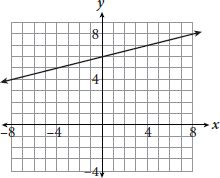Which of the following equations represents the line shown in the graph?

• A.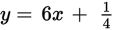• B.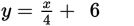• C.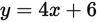• D.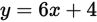Strategic Advice: When asked to match a graph to an equation, always start by examining the answer choices. Here, the equations are written in slope-intercept form (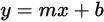), so find the slope of the line and its y-intercept and match them to m and b in the correct equation.
Getting to the Answer: The line crosses the vertical axis at (0, 6), making the y-intercept of the line 6, which means you can immediately eliminate A and D. To find the slope of the line, you can count the rise and the run from the y-intercept to the next point that lands on the intersection of two grid-lines, (4, 7), or you can use the slope formula. Either way, you'll find that the slope is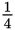, which makes (B) the correct answer.